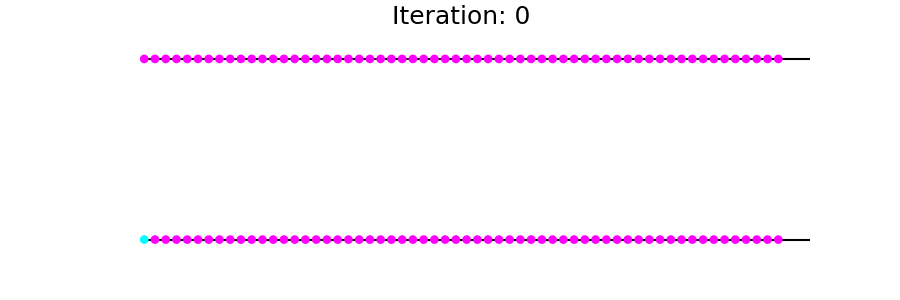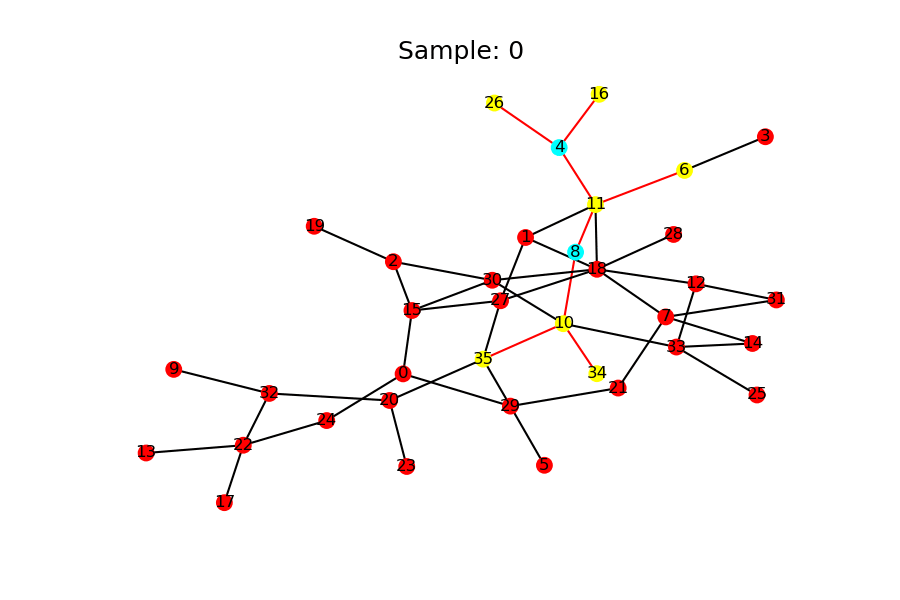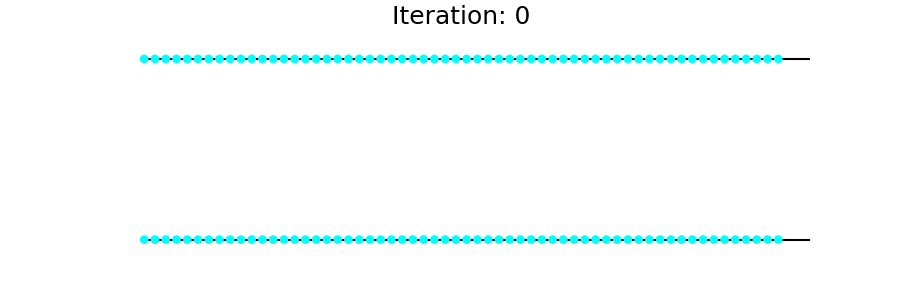Author: Gai Yu, Da Zheng, Quan Gan, Jinjing Zhou, Zheng Zhang

$\newcommand{\bfy}{\textbf{y}} \newcommand{\cale}{{\mathcal{E}}} \newcommand{\calg}{{\mathcal{G}}} \newcommand{\call}{{\mathcal{L}}} \newcommand{\caln}{{\mathcal{N}}} \newcommand{\calo}{{\mathcal{O}}} \newcommand{\calt}{{\mathcal{T}}} \newcommand{\calv}{{\mathcal{V}}} \newcommand{\until}{\text{until}\ }$

In this tutorial, you learn how to use the Deep Graph Library (DGL) with MXNet to implement the following:

Subgraph sampling is a technique to scale-up learning to gigantic graphs (for example, billions of nodes and edges). Subgraph sampling can apply to other algorithms, such as Graph convolution network and Relational graph convolution network.

Many algorithms for graph analytics are iterative procedures that end when a steady state is reached. Examples include PageRank or mean-field inference on Markov random fields.

### Flood-fill algorithm¶

A Flood-fill algorithm (or infection algorithm) can also be seen as a procedure. Specifically, the problem is that given a graph $$\calg = (\calv, \cale)$$ and a source node $$s \in \calv$$, you need to mark all nodes that can be reached from $$s$$. Let $$\calv = \{1, ..., n\}$$ and let $$y_v$$ indicate whether a node $$v$$ is marked. The flood-fill algorithm proceeds as follows.

\begin{split}\begin{alignat}{2} & y_s^{(0)} \leftarrow 1 \tag{0} \\ & y_v^{(0)} \leftarrow 0 \qquad && v \ne s \tag{1} \\ & y_v^{(t + 1)} \leftarrow \max_{u \in \caln (v)} y_u^{(t)} \qquad && \until \forall v \in \calv, y_v^{(t + 1)} = y_v^{(t)} \tag{2} \end{alignat}\end{split}

where $$\caln (v)$$ denotes the neighborhood of $$v$$, including $$v$$ itself.

The flood-fill algorithm first marks the source node $$s$$, and then repeatedly marks nodes with one or more marked neighbors until no node needs to be marked, that is, the steady state is reached.

### Flood-fill algorithm and steady-state operator¶

More abstractly, \begin{align} & y_v^{(0)} \leftarrow \text{constant} \\ & \bfy^{(t + 1)} \leftarrow \calt (\bfy^{(t)}) \qquad \until \bfy^{(t + 1)} = \bfy^{(t)} \tag{3} \end{align} where $$\bfy^{(t)} = (y_1^{(t)}, ..., y_n^{(t)})$$ and $$[\calt (\bfy^{(t)})]_v = \hat\calt (\{\bfy_u^{(t)} : u \in \caln (v)\})$$. In the case of the flood-fill algorithm, $$\hat\calt = \max$$. The condition “$$\until \bfy^{(t + 1)} = \bfy^{(t)}$$” in $$(3)$$ implies that $$\bfy^*$$ is the solution to the problem if and only if $$\bfy^* = \calt (\bfy^*)$$, that is $$\bfy^*$$ is steady under $$\calt$$. Thus we call $$\calt$$ the steady-state operator.

### Implementing a flood-fill algorithm¶

You can implement flood-fill in DGL with the following code.

import mxnet as mx
import os
import dgl

def T(g):
def message_func(edges):
return {'m': edges.src['y']}
def reduce_func(nodes):
# First compute the maximum of all neighbors...
m = mx.nd.max(nodes.mailbox['m'], axis=1)
# Then compare the maximum with the node itself.
# One can also add a self-loop to each node to avoid this
m = mx.nd.maximum(m, nodes.data['y'])
return {'y': m.reshape(m.shape, 1)}
g.update_all(message_func, reduce_func)
return g.ndata['y']


To run the algorithm, create a DGLGraph as in the example code here, consisting of two disjointed chains, each with ten nodes, and initialize it as specified in Eq. $$(0)$$ and Eq. $$(1)$$.

import networkx as nx

def disjoint_chains(n_chains, length):
path_graph = nx.path_graph(n_chains * length).to_directed()
for i in range(n_chains - 1):  # break the path graph into N chains
path_graph.remove_edge((i + 1) * length - 1, (i + 1) * length)
path_graph.remove_edge((i + 1) * length, (i + 1) * length - 1)
for n in path_graph.nodes:
return path_graph

N = 2    # the number of chains
L = 500 # the length of a chain
s = 0    # the source node
# The sampler (see the subgraph sampling section) only supports
y = mx.nd.zeros([g.number_of_nodes(), 1])
y[s] = 1
g.ndata['y'] = y


Out:

/home/ubuntu/prod-doc/readthedocs.org/user_builds/dgl/checkouts/0.5.x/python/dgl/base.py:45: DGLWarning: Recommend creating graphs by dgl.graph(data) instead of dgl.DGLGraph(data).
return warnings.warn(message, category=category, stacklevel=1)
/home/ubuntu/prod-doc/readthedocs.org/user_builds/dgl/checkouts/0.5.x/python/dgl/base.py:45: DGLWarning: Keyword arguments ['readonly'] are deprecated in v0.5, and can be safely removed in all cases.
return warnings.warn(message, category=category, stacklevel=1)


Now apply T to g until convergence. You can see that nodes reachable from s are gradually infected (marked).

while True:
prev_y = g.ndata['y']
next_y = T(g)
if all(prev_y == next_y):
break


The update procedure is visualized as follows:### Neural flood-fill algorithm¶

Next, you can design a neural network that simulates the flood-fill algorithm.

Instead of using $$\calt$$ to update the states of nodes, use

$$\calt_\Theta$$, a graph neural network (and $$\hat\calt_\Theta$$ instead of $$\hat\calt$$).

The state of a node $$v$$ is no longer a Boolean value

($$y_v$$), but, an embedding $$h_v$$ (a vector of some reasonable dimension, say, $$H$$).

You can also associate a feature vector $$x_v$$ with $$v$$. For

the flood-fill algorithm, simply use the one-hot encoding of a node’s ID as its feature vector, so that our algorithm can distinguish different nodes.

Only iterate $$T$$ times instead of iterating until the

After iteration, mark the nodes by passing the node embedding

$$h_v$$ into another neural network to produce a probability $$p_v$$ of whether the node is reachable.

Mathematically, \begin{align} & h_v^{(0)} \leftarrow \text{random embedding} \\ & h_v^{(t + 1)} \leftarrow \calt_\Theta (h_1^{(t)}, ..., h_n^{(t)}) \qquad 1 \leq t \leq T \tag{4} \end{align} where $$[\calt_\Theta (h_1^{(t)}, ..., h_n^{(t)})]_v = \hat\calt_\Theta (x_u, h_u^{(t)} : u \in \caln (v)\})$$. $$\hat\calt_\Theta$$ is a two layer neural network, as follows:

$\hat\calt_\Theta (\{x_u, h_u^{(t)} : u \in \caln (v)\}) = W_1 \sigma \left(W_2 \left[x_v, \sum_{u \in \caln (v)} \left[h_v, x_v\right]\right]\right)$

where $$[\cdot, \cdot]$$ denotes the concatenation of vectors, and $$\sigma$$ is a nonlinearity, e.g. ReLU. Essentially, for every node, $$\calt_\Theta$$ repeatedly gathers its neighbors’ feature vectors and embeddings, sums them up, and feeds the result along with the node’s own feature vector to a two layer neural network.

### Implementation of neural flood-fill¶

Like the naive algorithm, the neural flood-fill algorithm can be partitioned into a message_func (neighborhood information gathering) and a reduce_func ($$\hat\calt_\Theta$$). We define $$\hat\calt_\Theta$$ as a callable gluon.Block as in this example code.

import mxnet.gluon as gluon

def __init__(self, n_hidden, activation, **kwargs):
with self.name_scope():
self.dense1 = gluon.nn.Dense(n_hidden, activation=activation)
self.dense2 = gluon.nn.Dense(n_hidden)

def forward(self, g):
def message_func(edges):
x = edges.src['x']
h = edges.src['h']
return {'m' : mx.nd.concat(x, h, dim=1)}

def reduce_func(nodes):
m = mx.nd.sum(nodes.mailbox['m'], axis=1)
z = mx.nd.concat(nodes.data['x'], m, dim=1)
return {'h' : self.dense2(self.dense1(z))}

g.update_all(message_func, reduce_func)
return g.ndata['h']


In practice, Eq. $$(4)$$ may cause numerical instability. One solution is to update $$h_v$$ with a moving average, as follows:

$h_v^{(t + 1)} \leftarrow (1 - \alpha) h_v^{(t)} + \alpha \left[\calt_\Theta (h_0^{(t)}, ..., h_n^{(t)})\right]_v \qquad 0 < \alpha < 1$

Putting these together you have:

def update_embeddings(g, steady_state_operator):
prev_h = g.ndata['h']
g.ndata['h'] = (1 - alpha) * prev_h + alpha * next_h


The last step involves implementing the predictor.

class Predictor(gluon.Block):
def __init__(self, n_hidden, activation, **kwargs):
super(Predictor, self).__init__(**kwargs)
with self.name_scope():
self.dense1 = gluon.nn.Dense(n_hidden, activation=activation)
self.dense2 = gluon.nn.Dense(2)  ## binary classifier

def forward(self, h):
return self.dense2(self.dense1(h))


The predictor’s decision rule is just a decision rule for binary classification.

$\hat{y}_v = \text{argmax}_{i \in \{0, 1\}} \left[g_\Phi (h_v^{(T)})\right]_i \tag{5}$

where the predictor is denoted by $$g_\Phi$$ and $$\hat{y}_v$$ indicates whether the node $$v$$ is marked or not.

Our implementation can be further accelerated using DGL’s built-in functions, which maps the computation to more efficient sparse operators in the backend framework (e.g., MXNet/Gluon, PyTorch). Please see the Graph convolution network tutorial for more details.

### Efficient semi-supervised learning on graph¶

In this setting, you can observe the entire structure of one fixed graph as well as the feature vector of each node. However, you might only have access to the labels of some (very few) of the nodes. Train the neural flood-fill algorithm in this setting as well.

Initialize feature vectors 'x' and node embeddings 'h' first.

import numpy as np
import numpy.random as npr

n = g.number_of_nodes()
n_hidden = 16

g.ndata['x'] = mx.nd.eye(n, n)
g.ndata['y'] = mx.nd.concat(*[i * mx.nd.ones([L, 1], dtype='float32')
for i in range(N)], dim=0)
g.ndata['h'] = mx.nd.zeros([n, n_hidden])

r_train = 0.2  # the ratio of test nodes
n_train = int(r_train * n)
nodes_train = npr.choice(range(n), n_train, replace=True)
test_bitmap = np.ones(shape=(n))
test_bitmap[nodes_train] = 0
nodes_test = np.where(test_bitmap)


Unrolling the iterations in Eq. $$(4)$$, we have the following expression for updated node embeddings:

$h_v^{(T)} = \calt_\Theta^T (h_1^{(0)}, ..., h_n^{(0)}) \qquad v \in \calv \tag{6}$

where $$\calt_\Theta^T$$ means applying $$\calt_\Theta$$ for $$T$$ times. These updated node embeddings are fed to $$g_\Phi$$ as in Eq. $$(5)$$. These steps are fully differentiable and the neural flood-fill algorithm can thus be trained in an end-to-end fashion. Denoting the binary cross-entropy loss by $$l$$, you have a loss function in the following form:

$\call (\Theta, \Phi) = \frac1{\left|\calv_y\right|} \sum_{v \in \calv_y} l \left(g_\Phi \left(\left[\calt_\Theta^T (h_1^{(0)}, ..., h_n^{(0)})\right]_v \right), y_v\right) \tag{7}$

After computing $$\call (\Theta, \Phi)$$, you can update $$\Theta$$ and $$\Phi$$ using the gradients $$\nabla_\Theta \call (\Theta, \Phi)$$ and $$\nabla_\Phi \call (\Theta, \Phi)$$. One problem with Eq. $$(7)$$ is that computing $$\nabla_\Theta \call (\Theta, \Phi)$$ and $$\nabla_\Phi \call (\Theta, \Phi)$$ requires back-propagating $$T$$ times through $$\calt_\Theta$$, which may be slow in practice. So, adopt the following steady-state loss function, which only incorporates the last node embedding update in back-propagation:

$\call_\text{SteadyState} (\Theta, \Phi) = \frac1{\left|\calv_y\right|} \sum_{v \in \calv_y} l \left(g_\Phi \left(\left[\calt_\Theta (h_1^{(T - 1)}, ..., h_n^{(T - 1)})\right]_v, y_v\right)\right) \tag{8}$

The following implements one step of training with $$\call_\text{SteadyState}$$. Note that g in the following is $$\calg_y$$ instead of $$\calg$$.

def fullgraph_update_parameters(g, label_nodes, steady_state_operator, predictor, trainer):
n = g.number_of_nodes()
z = predictor(g.ndata['h'][label_nodes])
y = g.ndata['y'].reshape(n)[label_nodes]  # label
loss = mx.nd.softmax_cross_entropy(z, y)
loss.backward()
trainer.step(n)  # divide gradients by the number of labelled nodes
return loss.asnumpy()


You are now ready to implement the training procedure, which is in two phases.

• The first phase updates node embeddings several times using $$\calt_\Theta$$ to attain an approximately steady state

• The second phase trains $$\calt_\Theta$$ and $$g_\Phi$$ using this steady state.

You update the node embeddings of $$\calg$$ instead of $$\calg_y$$ only. The reason lies in the semi-supervised learning setting. To do inference on $$\calg$$, you need node embeddings on $$\calg$$ instead of on $$\calg_y$$ only.

def train(g, label_nodes, steady_state_operator, predictor, trainer):
# first phase
# second phase
predictor, trainer)
return loss


## Scaling up with stochastic subgraph training¶

The computation time per update is linear to the number of edges in a graph. If we have a gigantic graph with billions of nodes and edges, the update function would be inefficient.

A possible improvement draws an analogy from mini-batch training on large datasets. Instead of computing gradients on the entire graph, only consider some subgraphs randomly sampled from the labelled nodes. Mathematically, you have the following loss function:

$\call_\text{StochasticSteadyState} (\Theta, \Phi) = \frac1{\left|\calv_y^{(k)}\right|} \sum_{v \in \calv_y^{(k)}} l \left(g_\Phi \left(\left[\calt_\Theta (h_1, ..., h_n)\right]_v\right), y_v\right)$

where $$\calv_y^{(k)}$$ is the subset sampled for iteration $$k$$.

In this training procedure, you also update node embeddings only on sampled subgraphs, which is perhaps not surprising if you know stochastic fixed-point iteration.

### Neighbor sampling¶

You can use neighbor sampling as a subgraph sampling strategy. Neighbor sampling traverses small neighborhoods from seed nodes with breadth first search. For each newly sampled node, a small subset of neighboring nodes are sampled and added to the subgraph along with the connecting edges, unless the node reaches the maximum of $$k$$ hops from the seeding node.

The following shows neighbor sampling with two seed nodes at a time, a maximum of two hops, and a maximum of three neighboring nodes.DGL supports very efficient subgraph sampling natively. This helps users scale algorithms to large graphs. Currently, DGL provides the NeighborSampler() API, which returns a subgraph iterator that samples multiple subgraphs at a time with neighbor sampling.

The following code demonstrates how to use the NeighborSampler to sample subgraphs, and stores the seed nodes of the subgraph in each iteration:

nx_G = nx.erdos_renyi_graph(36, 0.06)
sampler = dgl.contrib.sampling.NeighborSampler(
G, 2, 3, num_hops=2, shuffle=True)
seeds = []
for subg in sampler:
seeds.append(subg.layer_parent_nid(-1))

Traceback (most recent call last):
File "/home/ubuntu/.pyenv/versions/miniconda3-latest/lib/python3.7/site-packages/sphinx_gallery/gen_rst.py", line 482, in _memory_usage
out = func()
File "/home/ubuntu/.pyenv/versions/miniconda3-latest/lib/python3.7/site-packages/sphinx_gallery/gen_rst.py", line 467, in __call__
exec(self.code, self.globals)
File "/home/ubuntu/prod-doc/readthedocs.org/user_builds/dgl/checkouts/0.5.x/tutorials/models/1_gnn/8_sse_mx.py", line 426, in <module>
G, 2, 3, num_hops=2, shuffle=True)
File "/home/ubuntu/prod-doc/readthedocs.org/user_builds/dgl/checkouts/0.5.x/python/dgl/contrib/sampling/sampler.py", line 320, in __init__
assert g.is_readonly, "NeighborSampler doesn't support mutable graphs. " + \
AssertionError: NeighborSampler doesn't support mutable graphs. Please turn it into an immutable graph with DGLGraphStale.readonly


### Sample training with DGL¶

The code illustrates the training process in mini-batches.

class SubgraphSteadyStateOperator(gluon.Block):
def __init__(self, n_hidden, activation, **kwargs):
with self.name_scope():
self.dense1 = gluon.nn.Dense(n_hidden, activation=activation)
self.dense2 = gluon.nn.Dense(n_hidden)

def forward(self, subg):
def message_func(edges):
x = edges.src['x']
h = edges.src['h']
return {'m' : mx.nd.concat(x, h, dim=1)}

def reduce_func(nodes):
m = mx.nd.sum(nodes.mailbox['m'], axis=1)
z = mx.nd.concat(nodes.data['x'], m, dim=1)
return {'h' : self.dense2(self.dense1(z))}

subg.block_compute(0, message_func, reduce_func)
return subg.layers[-1].data['h']

n = subg.layer_size(-1)
z = predictor(subg.layers[-1].data['h'])
y = subg.layers[-1].data['y'].reshape(n)  # label
loss = mx.nd.softmax_cross_entropy(z, y)
loss.backward()
trainer.step(n)  # divide gradients by the number of labelled nodes
return loss.asnumpy()

# Note that we are only updating the embeddings of seed nodes here.
# The reason is that only the seed nodes have ample information
# from neighbors, especially if the subgraph is small (e.g. 1-hops)
prev_h = g.layers[-1].data['h']
g.layers[-1].data['h'] = (1 - alpha) * prev_h + alpha * next_h

def train_on_subgraphs(g, label_nodes, batch_size,
# To train SSE, we create two subgraph samplers with the
# NeighborSampler API for each phase.

# The first phase samples from all vertices in the graph.
sampler = dgl.contrib.sampling.NeighborSampler(
g, batch_size, g.number_of_nodes(), num_hops=1)
sampler_iter = iter(sampler)

# The second phase only samples from labeled vertices.
sampler_train = dgl.contrib.sampling.NeighborSampler(
g, batch_size, g.number_of_nodes(), seed_nodes=label_nodes, num_hops=1)
sampler_train_iter = iter(sampler_train)

subg = next(sampler_iter)
# Currently, subgraphing does not copy or share features
# automatically.  Therefore, we need to copy the node
# embeddings of the subgraph from the parent graph with
# copy_from_parent() before computing...
subg.copy_from_parent()
# ... and copy them back to the parent graph.
g.ndata['h'][subg.layer_parent_nid(-1)] = subg.layers[-1].data['h']
try:
subg = next(sampler_train_iter)
except:
break
# Again we need to copy features from parent graph
subg.copy_from_parent()
loss = update_parameters_subgraph(subg, steady_state_operator, predictor, trainer)
# We don't need to copy the features back to parent graph.
return loss


You can also define a helper function that reports prediction accuracy.

def test(g, test_nodes, predictor):
z = predictor(g.ndata['h'][test_nodes])
y_bar = mx.nd.argmax(z, axis=1)
y = g.ndata['y'].reshape(n)[test_nodes]
accuracy = mx.nd.sum(y_bar == y) / len(test_nodes)
return accuracy.asnumpy(), z


Some routine preparations for training.

lr = 1e-3
activation = 'relu'

predictor = Predictor(n_hidden, activation)
predictor.initialize()
params.update(predictor.collect_params())
trainer = gluon.Trainer(params, 'adam', {'learning_rate' : lr})


Now train it. As before, nodes reachable from $$s$$ are gradually infected, except that in the background is a neural network.

n_epochs = 35
alpha = 0.1
batch_size = 64

y_bars = []
for i in range(n_epochs):
loss = train_on_subgraphs(g, nodes_train, batch_size, subgraph_steady_state_operator,
predictor, trainer)

accuracy_train, _ = test(g, nodes_train, predictor)
accuracy_test, z = test(g, nodes_test, predictor)
print("Iter {:05d} | Train acc {:.4} | Test acc {:.4f}".format(i, accuracy_train, accuracy_test))
y_bar = mx.nd.argmax(z, axis=1)
y_bars.append(y_bar)In this tutorial, you used a very small example graph to demonstrate the subgraph training for easy visualization. Subgraph training actually helps you scale to gigantic graphs. For instance, scaling SSE to a graph with 50 million nodes and 150 million edges in a single P3.8x large instance, and one epoch, only takes about 160 seconds.

For full examples, see Benchmark SSE on multi-GPUs on Github.

Total running time of the script: ( 0 minutes 10.564 seconds)

Gallery generated by Sphinx-Gallery